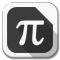Find the Inverse of a Function - Part I
111 views · Feb 20, 2022
Visit Channel:```A tutorial on to find the inverse of a function given by a formula. From www.analyzemath.com

http://www.analyzemath.com/stepbystep_mathworksheets/functions/inverse_square_root.html

http://analyzemath.com/stepbystep_mathworksheets/functions/inverse_linear.html

http://analyzemath.com/stepbystep_mathworksheets/functions/inverse_cubic.html```

##### Latest Videos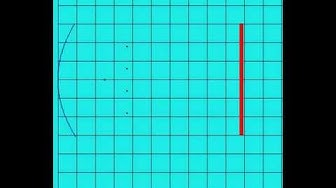1:07Parabolic Reflector form http://www.analyzemath.com
5 views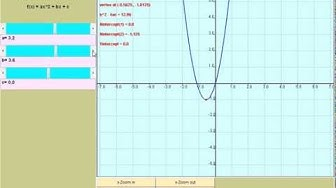2:54120 views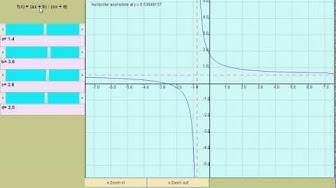1:47Rational Functions from www.analyzemath.com
49 views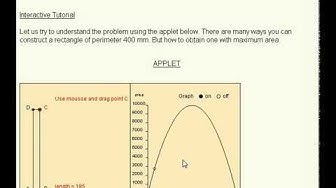2:24Maximum Area of Rectangle form www.analyzemath.com
10 views7:23Find the Domain of Rational Functions
99 views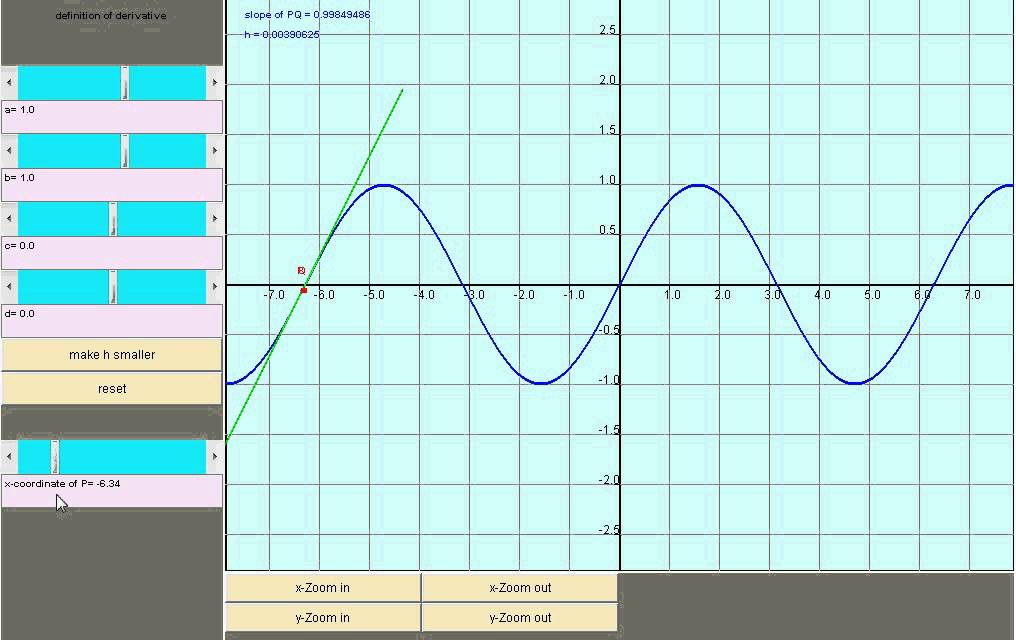2:24First Derivative Applet from www.analyzemath.com
580 views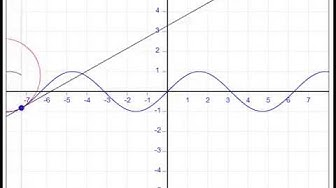0:57osculating circle and second derivative
2 views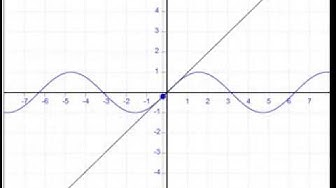1:01Graphical interpretation of First Derivative
1K views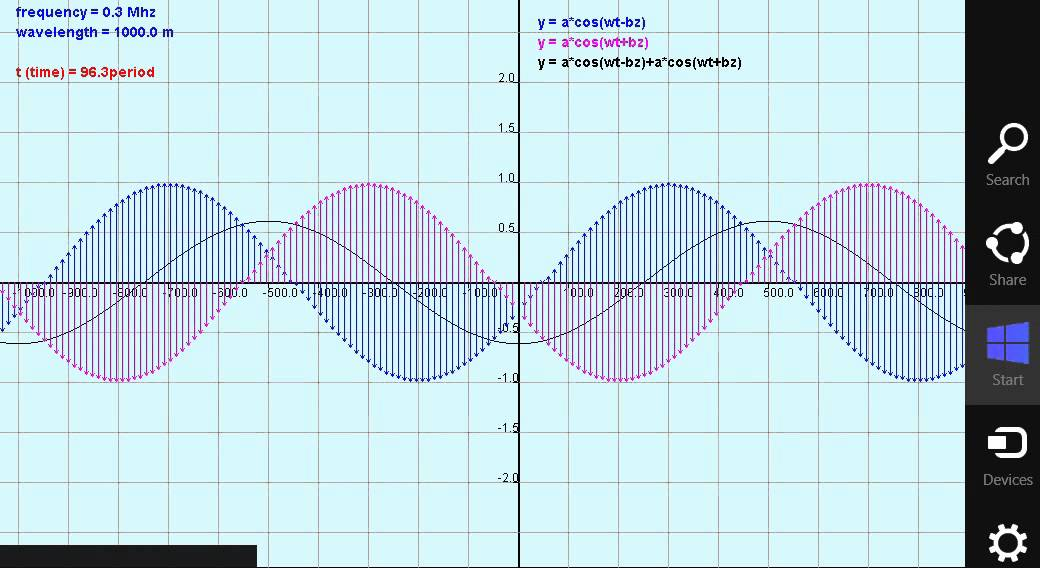0:49standing waves
54 views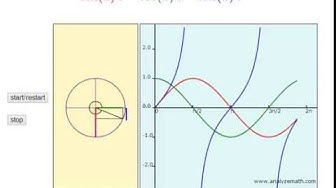1:05unit circle and trigonometric functions
0 views